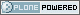# Neuroimaging Informatics Technology Initiative

##### Personal tools
You are here: Home » Quaternion representation

# Quaternion representation

## quatern_*, qoffset_*

```
QUATERNION REPRESENTATION OF ROTATION MATRIX (METHOD 2)
-------------------------------------------------------
The orientation of the (x,y,z) axes relative to the (i,j,k) axes
in 3D space is specified using a unit quaternion [a,b,c,d], where
a*a+b*b+c*c+d*d=1.  The (b,c,d) values are all that is needed, since
we require that a = sqrt(1.0-(b*b+c*c+d*d)) be nonnegative.  The (b,c,d)
values are stored in the (quatern_b,quatern_c,quatern_d) fields.

The quaternion representation is chosen for its compactness in
representing rotations. The (proper) 3x3 rotation matrix that
corresponds to [a,b,c,d] is

[ a*a+b*b-c*c-d*d   2*b*c-2*a*d       2*b*d+2*a*c     ]
R = [ 2*b*c+2*a*d       a*a+c*c-b*b-d*d   2*c*d-2*a*b     ]
[ 2*b*d-2*a*c       2*c*d+2*a*b       a*a+d*d-c*c-b*b ]

[ R11               R12               R13             ]
= [ R21               R22               R23             ]
[ R31               R32               R33             ]

If (p,q,r) is a unit 3-vector, then rotation of angle h about that
direction is represented by the quaternion

[a,b,c,d] = [cos(h/2), p*sin(h/2), q*sin(h/2), r*sin(h/2)].

Requiring a >= 0 is equivalent to requiring -Pi <= h <= Pi.  (Note that
[-a,-b,-c,-d] represents the same rotation as [a,b,c,d]; there are 2
quaternions that can be used to represent a given rotation matrix R.)
To rotate a 3-vector (x,y,z) using quaternions, we compute the
quaternion product

[0,x',y',z'] = [a,b,c,d] * [0,x,y,z] * [a,-b,-c,-d]

which is equivalent to the matrix-vector multiply

[ x' ]     [ x ]
[ y' ] = R [ y ]   (equivalence depends on a*a+b*b+c*c+d*d=1)
[ z' ]     [ z ]

Multiplication of 2 quaternions is defined by the following:

[a,b,c,d] = a*1 + b*I + c*J + d*K
where
I*I = J*J = K*K = -1 (I,J,K are square roots of -1)
I*J =  K    J*K =  I    K*I =  J
J*I = -K    K*J = -I    I*K = -J  (not commutative!)
For example
[a,b,0,0] * [0,0,0,1] = [0,0,-b,a]
since this expands to
(a+b*I)*(K) = (a*K+b*I*K) = (a*K-b*J).

The above formula shows how to go from quaternion (b,c,d) to
rotation matrix and direction cosines.  Conversely, given R,
we can compute the fields for the NIFTI-1 header by

a = 0.5  * sqrt(1+R11+R22+R33)    (not stored)
b = 0.25 * (R32-R23) / a       => quatern_b
c = 0.25 * (R13-R31) / a       => quatern_c
d = 0.25 * (R21-R12) / a       => quatern_d

If a=0 (a 180 degree rotation), alternative formulas are needed.
See the nifti1_io.c function mat44_to_quatern() for an implementation
of the various cases in converting R to [a,b,c,d].

Note that R-transpose (= R-inverse) would lead to the quaternion
[a,-b,-c,-d].

The choice to specify the qoffset_x (etc.) values in the final
coordinate system is partly to make it easy to convert DICOM images to
this format.  The DICOM attribute "Image Position (Patient)" (0020,0032)
stores the (Xd,Yd,Zd) coordinates of the center of the first voxel.
Here, (Xd,Yd,Zd) refer to DICOM coordinates, and Xd=-x, Yd=-y, Zd=z,
where (x,y,z) refers to the NIFTI coordinate system discussed above.
(i.e., DICOM +Xd is Left, +Yd is Posterior, +Zd is Superior,
whereas +x is Right, +y is Anterior  , +z is Superior. )
Thus, if the (0020,0032) DICOM attribute is extracted into (px,py,pz), then
qoffset_x = -px   qoffset_y = -py   qoffset_z = pz
is a reasonable setting when qform_code=NIFTI_XFORM_SCANNER_ANAT.

That is, DICOM's coordinate system is 180 degrees rotated about the z-axis
from the neuroscience/NIFTI coordinate system.  To transform between DICOM
and NIFTI, you just have to negate the x- and y-coordinates.

The DICOM attribute (0020,0037) "Image Orientation (Patient)" gives the
orientation of the x- and y-axes of the image data in terms of 2 3-vectors.
The first vector is a unit vector along the x-axis, and the second is
along the y-axis.  If the (0020,0037) attribute is extracted into the
value (xa,xb,xc,ya,yb,yc), then the first two columns of the R matrix
would be
[ -xa  -ya ]
[ -xb  -yb ]
[  xc   yc ]
The negations are because DICOM's x- and y-axes are reversed relative
to NIFTI's.  The third column of the R matrix gives the direction of
displacement (relative to the subject) along the slice-wise direction.
This orientation is not encoded in the DICOM standard in a simple way;
DICOM is mostly concerned with 2D images.  The third column of R will be
either the cross-product of the first 2 columns or its negative.  It is
possible to infer the sign of the 3rd column by examining the coordinates
in DICOM attribute (0020,0032) "Image Position (Patient)" for successive
slices.  However, this method occasionally fails for reasons that I
(RW Cox) do not understand.

```
Created by Alex Clark